# Input-Output Relationships of Transmitters

Have you ever had that actually forget something simple? And generally forgotten it was in the form like a formula. For example, the formula to convert temperature unit of degrees Celsius to degrees Fahrenheit. Generally we use the formula:

#### {(9/5) * x + 32 ° F}

Where “x”  is the temperature in Deg C

So as to know how to calculate ° F of the temperature of 50 ° C, if using the above formula to

{(9/5) * x + 32 ° F} = {(9/5) * 50 ° F} + 32 = 122 ° F

Easy enough right? Yes, if we memorized formula. The problem is if we completely forgot the formula.

### The calculation of input-output relationships of transmitter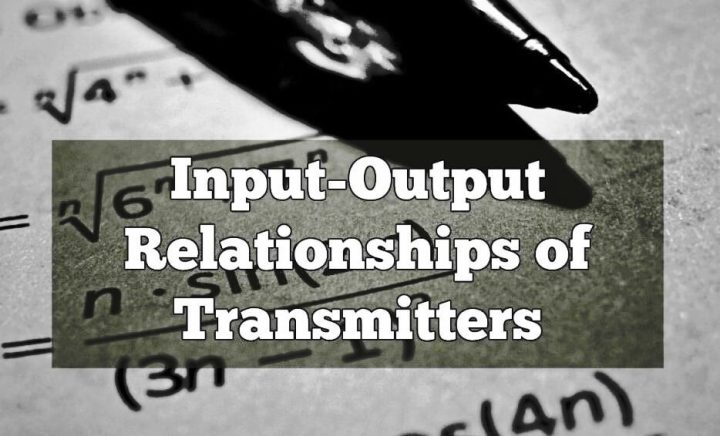Something similar may happen when we’re doing calibration, like a pressure transmitter. For example, with an input pressure of 0-300 PSI and 4-20mA output.

If we use the method of 5-point calibration (0%, 25%, 50%, 75% and 100%), it may be quite easy for us, it should be how we put pressure on the transmitter at the time of testing at 50% (12mA). But it is different if we verify the pressure transmitter is already on-line with different process value.

For example, we find the pressure on the input side of the transmitter is 235 PSI, then what is the pressure transmitter output signal?

Back to the issue of conversion of temperature. If we forget the formula, we can actually do the conversion without having memorized the formula, the condition we know the upper limit and lower limit for the second unit. Then, using the equation of general comparison, we can create their own respective formula.

We know that the units Celsius has a measuring range (measuring scale for the calculation) between 0-100 ° C and Fahrenheit 32-212 ° F. So if asked, 50 ° C was how ° F, then: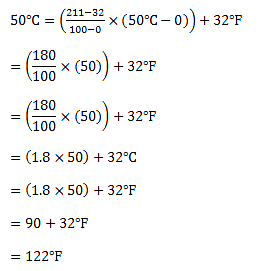See calculation above, it may be longer than we memorize the formula, here we do not try to memorize formulas, but understand the concept, because the first formula above were true simplification of calculations a second.

The concept of how it mean? This calculation is a calculation of comparison, to compare two different scales.

Let’s agree that:

x ° C = value ° C

C min   = minimum scale ° C = 0 ° C

C max  = maximum scale ° C = 100 ° C

x ° F = value ° F

F min  = minimum scale ° F = 32 ° F

F max maximum scale ° F = 212 ° F

thus: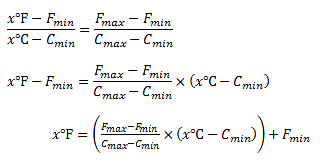Using the above equation: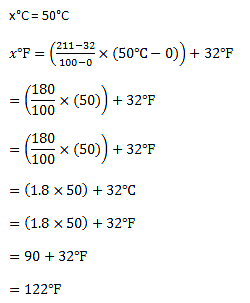This applies to calculate the output of a transmitter at the time of a particular input. For example, as the above example, we have a pressure transmitter with 0-300 PSI pressure input range and output 4-20mA.

Let’s agree:

x in   = value transmitter input (235PSI)

In min   = scale of minimum input transmitter = 0PSI

In max   = maximum scale transmitter input = 300PSI

x out = transmitter output value (mA)

Out min   = minimum scale transmitter output = 4mA

Out max = maximum scale = 20mA transmitter output

With reference to the equation of comparison, the value of 235PSI input transmitter can be calculated:By understanding the concept of the above, we can make a generic equation for the relation between the input and output of a transmitter as follows:Where:

x dit   = value in question (want to know)

dit min   = minimum scale range in question

dit max   = maximum scale range in question = 300PSI

x dik = value unknown

dik min   = minimum scale of a range of known

dik max = maximum scale of the known range

Example:

#### A temperature transmitter with an input range +50 to 200 ° F and output range is 4-20mA. what is the output if input temperature is 61 ° F:

dit min   = 4

dit max   = 20

x dik = 61

dik min   = 50

dik max  = 200

Then: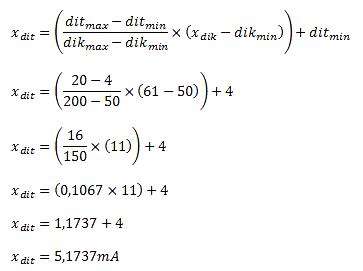Thus, the input temperature of 61 ° F, the transmitter output is 5.1737 mA

Thus, a generic formula for calculating the relationship of input and output transmitter or vice versa, can use:##### A temperature transmitter with input range -25 to 100 ° C and output 4-20mA range, Transmitter output is 17.5 mA, and find out temperature reading ?

Try to calculate the answer using above method:

#### Articles You May Like :

Level Control Loop Tuning

Percentage to Process Variable

Transmitter Process Variable

Control Valve Cv calculation

Don't Miss Our Updates
Be the first to get exclusive content straight to your email.
We promise not to spam you. You can unsubscribe at any time.

### 6 thoughts on “Input-Output Relationships of Transmitters”

1.Was very useful. Can’t believe how we think of simple things as complicated ones. Thanks.

2.Thanks sir , you are great !!! your informations are powerfull.

Please explain how to calibrate FF temperature and pressure transmitter using field communicator

3.Thank u sir.

4.may I know what is the answer sir?

5.•